DL框架之Tensorflow：深度学习框架Tensorflow的简介、安装、使用方法之详细攻略（三）

+关注继续查看

2、相关概念

1、张量(tensor)

TensorFlow 中的核心数据单位是张量（tensor）。一个张量由一组形成阵列（任意维数）的原始值组成。张量的阶(rank)是它的维数，而它的形状(shape)是一个整数元组，指定了阵列每个维度的长度。以下是张量值的一些示例：

3.    # a rank 0 tensor; a scalar with shape [],

[1., 2., 3.] # a rank 1 tensor; a vector with shape 

[[1., 2., 3.], [4., 5., 6.]] # a rank 2 tensor; a matrix with shape [2, 3]

[[[1., 2., 3.]], [[7., 8., 9.]]] # a rank 3 tensor with shape [2, 1, 3]

TensorFlow 使用numpy数组来表示张量值。

tf.Tensor 具有以下属性：

张量中的每个元素都具有相同的数据类型，且该数据类型一定是已知的。形状，即张量的维数和每个维度的大小，可能只有部分已知。如果其输入的形状也完全已知，则大多数操作会生成形状完全已知的张量，但在某些情况下，只能在执行图时获得张量的形状。

(1)、阶(rank)和形状(shape)

tf.Tensor 对象的阶是它本身的维数。阶的同义词包括：秩、等级或n 维。请注意，TensorFlow 中的阶与数学中矩阵的阶并不是同一个概念。如下表所示，TensorFlow 中的每个阶都对应一个不同的数学实例：

张量的形状是每个维度中元素的数量。TensorFlow 在图的构建过程中自动推理形状。这些推理的形状可能具有已知或未知的阶。如果阶已知，则每个维度的大小可能已知或未知。TensorFlow 文件编制中通过三种符号约定来描述张量维度：阶，形状和维数。下表阐述了三者如何相互关联：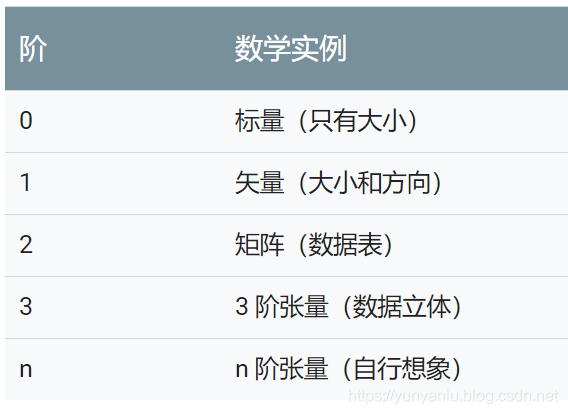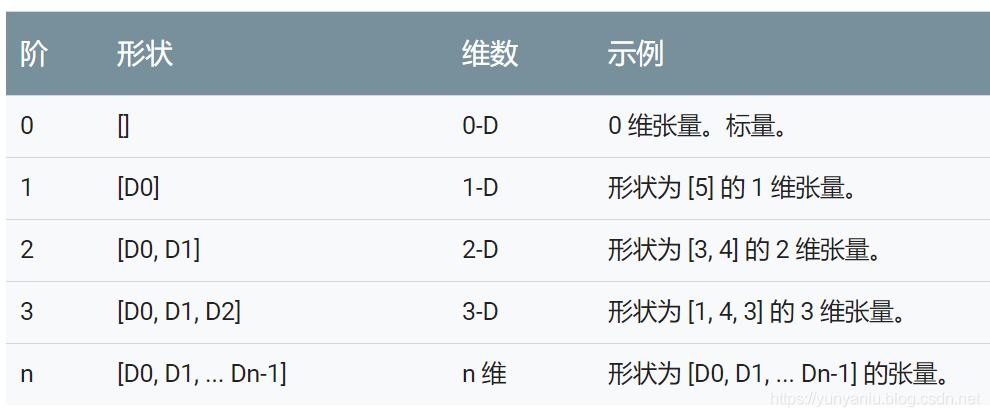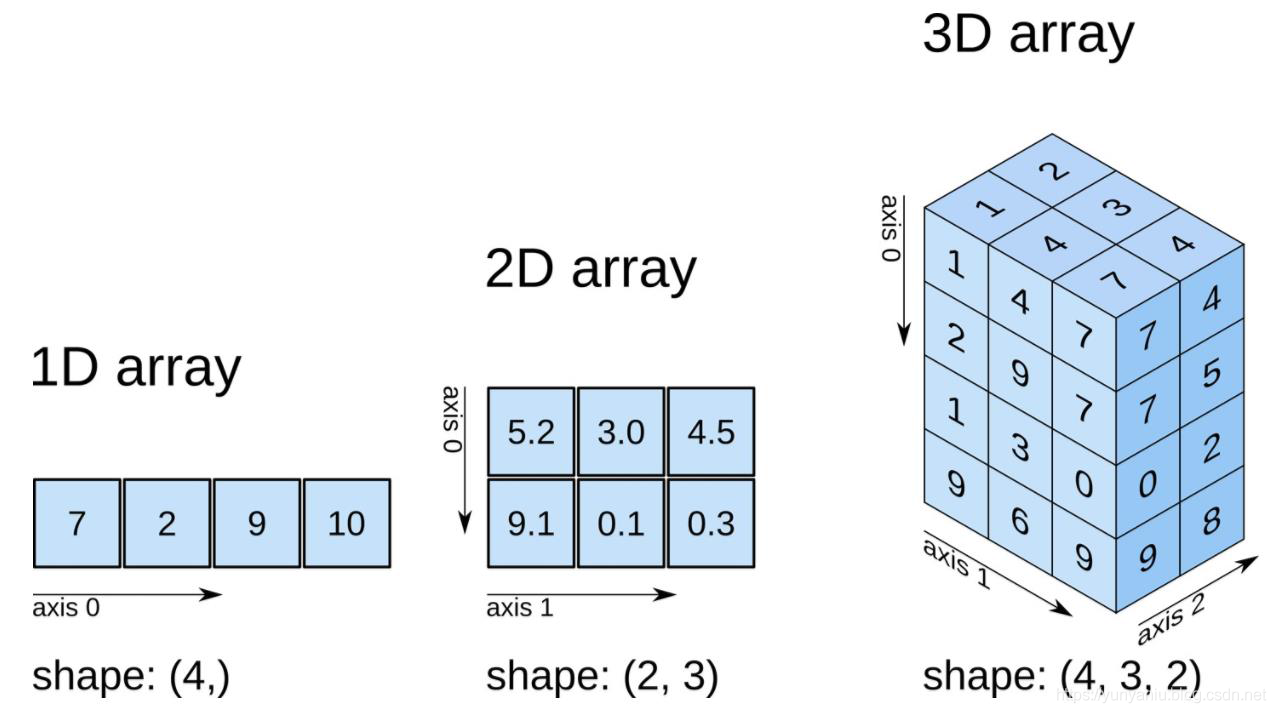TensorFlow的使用方法

TF学习：Tensorflow基础案例、经典案例集合——基于python编程代码的实现

1、基础函数

sess.run()

#当我们构建完图后，需要在一个会话中启动图，启动的第一步是创建一个Session对象。  为了取回（Fetch）操作的输出内容, 可以在使用 Session 对象的 run()调用执行图时，传入一些 tensor, 这些 tensor 会帮助你取回结果。那么调用sess.run()的时候，ensorflow并没有计算整个图，只是计算了与想要fetch 的值相关的部分。

2、输出tensorflow的版本号

import tensorflow as tf

print('输出tensorflow的版本：',tf.__version__)

案例应用

DL框架之Tensorflow：Tensorflow中常用函数的简介、使用方法之详细攻略

1、使用常量和占位符进行计算

#使用常量和占位符进行计算：其中 y_1 的计算过程使用占位符，而 y_2 的计算过程使用常量

w1=tf. Variable (tf.random_normal([ 1 , 2 ],stddev= 1 ,seed= 1 ))  #因为需要重复输入x，而每建一个x就会生成一个结点，计算图的效率会低。所以使用占位符

x=tf.placeholder(tf.float32,shape=( 1 , 2 ))

x1=tf.constant([[ 0.7 , 0.9 ]])

a=x+w1

b=x1+w1

sess=tf. Session ()

sess.run(tf.global_variables_initializer())  #运行y时将占位符填上，feed_dict为字典，变量名不可变

y_1=sess.run(a,feed_dict={x:[[ 0.7 , 0.9 ]]})

y_2=sess.run(b)

print (y_1)

print (y_2)

sess.close

2、输出w、b和计算两个数值相乘

import tensorflow as tf

import numpy as np

W = tf.Variable(np.arange(6).reshape((2, 3)), dtype=tf.float32, name="weights")

b = tf.Variable(np.arange(3).reshape((1, 3)), dtype=tf.float32, name="biases")

saver = tf.train.Saver()

with tf.Session() as sess:

saver.restore(sess, "niu/save_net.ckpt")

print("weights:", sess.run(W))

print("biases:", sess.run(b))

import tensorflow as tf

#构建一个计算图

a=tf.constant([1.0, 2.0])

b=tf.constant([3.0, 4.0])

c=a*b

#T1、传统的方法，先构建再释放

sess=tf.Session()   #构建一个Session

print(sess.run(c))  #把计算图放到session里，并运行得到结果

sess.close()        #关闭session释放资源

#T2、with语句的方法，先构建再释放

with tf.Session() as sess:

print(sess.run(c))  #把计算图放到session里，并运行得到结果

3、ML之LoR：利用LoR算法(tensorflow)对mnist数据集实现手写数字识别

import tensorflow as tf

import numpy as np

from tensorflow.examples.tutorials.mnist import input_data

#1、定义数据集：mnist

trainimg       =  mnist.train.images

trainimg_label =  mnist.train.labels

testimg        =  mnist.test.images

testimg_label  =  mnist.test.labels

print(trainimg_label) #输出第一行label值

#2、定义x、y、w、b：tf.placeholder占位符、tf.Variable变量

x = tf.placeholder("float",[None,784])  # [None,784]样本的个数（无限大），每个样本的特征（784个像素点）

y = tf.placeholder("float",[None,10])   #样本的类别（10个）

W = tf.Variable(tf.zeros([784,10]))     #每个特征（784个像素点）对应输出10个分类值

b = tf.Variable(tf.zeros())

#3、模型预测：LoR(softmax多分类)

#3.1、定义计算损失：actv、cost

actv = tf.nn.softmax(tf.matmul(x,W)+b)                                     #计算属于正确类别的概率值

cost = tf.reduce_mean(-tf.reduce_sum(y*tf.log(actv),reduction_indices=1))  #计算损失值（预测值与真实值间的均方差）

#3.2、定义模型训练：learning_rate、optm：

#(1)、采用GD优化参数w、b，最小化损失值

learning_rate=0.01

# optm = optimizer.minimize(cost)                                        #最小化损失值

#3.3、定义模型的pred、accr

pred =    tf.equal(tf.argmax(actv,1),tf.argmax(y,1))  #预测值：equal返回的值是布尔类型，argmax返回矩阵中最大元素的索引，0，代表列方向；1代表行方向

accr =    tf.reduce_mean(tf.cast(pred,"float"))       #准确率，cast进行类型转化 （true为1，false为0）

#3.4、定义模型训练参数init_op、train_epochs、batch_size、display_step

init_op = tf.global_variables_initializer()       #初始化所有variables 的op

train_epochs = 50   #将所有样本迭代50次

batch_size = 100       #每次迭代选择样本的个数

display_step =5        #每进行5个epoch进行一次展示

#3.5、运行模型tf.Session()

with tf.Session() as sess:             #在session中启动graph

sess.run(init_op)                      #启动运行使用variables的op

for epoch in range(train_epochs):

#(1)、定义avg_cost、num_batch

avg_cost =0.0  #初始化损失值

num_batch = int(mnist.train.num_examples/batch_size)

#(2)、for循环实现num_batch批量训练

for i in range(num_batch):

batch_xs, batch_ys = mnist.train.next_batch(batch_size)  #以batch为单位逐次进行

sess.run(optm,feed_dict={x: batch_xs,y: batch_ys})       #给x，y赋值

feeds={x: batch_xs,y: batch_ys}

avg_cost +=sess.run(cost,feed_dict= feeds)/num_batch

#(3)、if判断实现每轮结果输出：输出每轮(5个epoch)的cost、trian_acc、test_acc

if epoch %  display_step == 0:

feeds_train = {x: batch_xs,y: batch_ys}

feeds_test  = {x:mnist.test.images,y: mnist.test.labels}

train_acc   =  sess.run(accr,feed_dict= feeds_train)

test_acc    =  sess.run(accr,feed_dict= feeds_test)

print("Epoch: %03d/%03d cost:%.9f trian_acc: %.3f test_acc: %.3f"

% (epoch,train_epochs,avg_cost,train_acc,test_acc))

print("Done")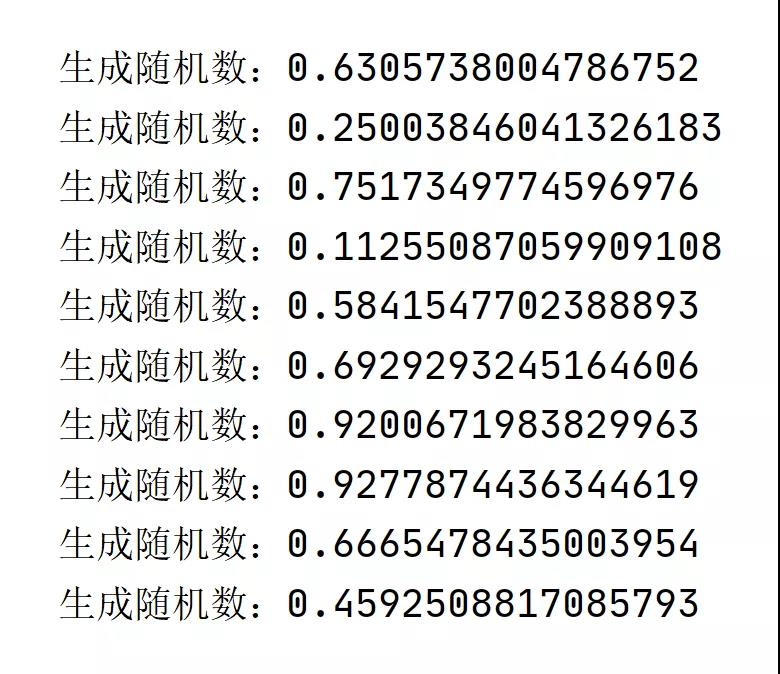Java生成随机数的4种方式，以后就用它了!（7）
Java生成随机数的4种方式，以后就用它了!（7）
5 0Python3使用RSA分段加密解密

6 0python中循环的技巧

7 0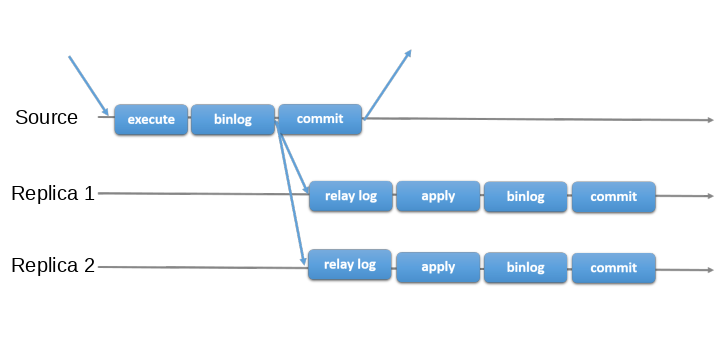MySQL MGR模式介绍
MGR是Mysql Group Replication(组复制)的缩写，Mysql5.7之后是以一个Mysql插件的形式集成在Mysql中，用于创建可伸缩、高可用、可容错的复制架构，是Mysql集群的一种形式
10 0Python合并pdf方法
Python合并pdf方法
4 0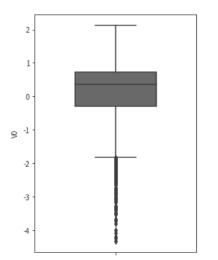9 0python中对切片的理解

7 0mysql总结
mysql总结
25 0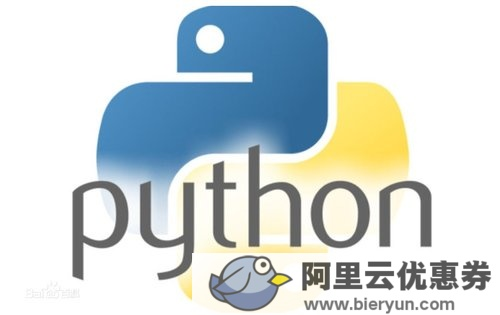python测试框架-pytest
python测试框架-pytest 一、pytest 介绍、运行、参数化和数据驱动、Fixture pytest安装与介绍 官网 : pip install -U pytest 查看版本号：pytest --version 为何选择pytest 兼容unittest 定制化插件开发 pycharm 配置github VSC--Git--Remotes... pycharm pytest 配置 settings--搜索pytest--Python integrated Tools--testing--选择pytest:根据黄色叹号fix安装pytest安装到环境
22 0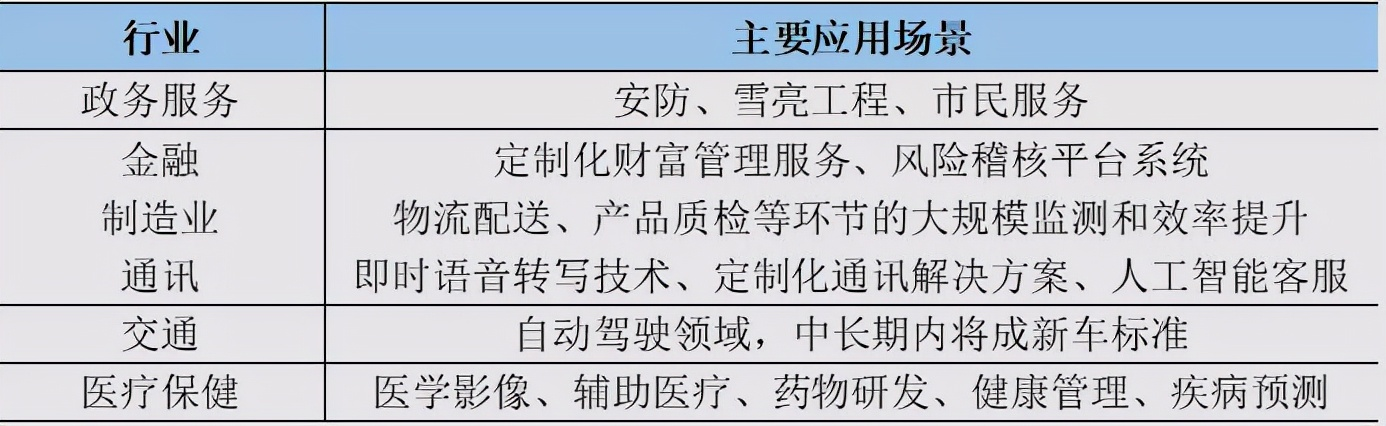12 0

1701

0

《2021云上架构与运维峰会演讲合集》

《零基础CSS入门教程》

《零基础HTML入门教程》